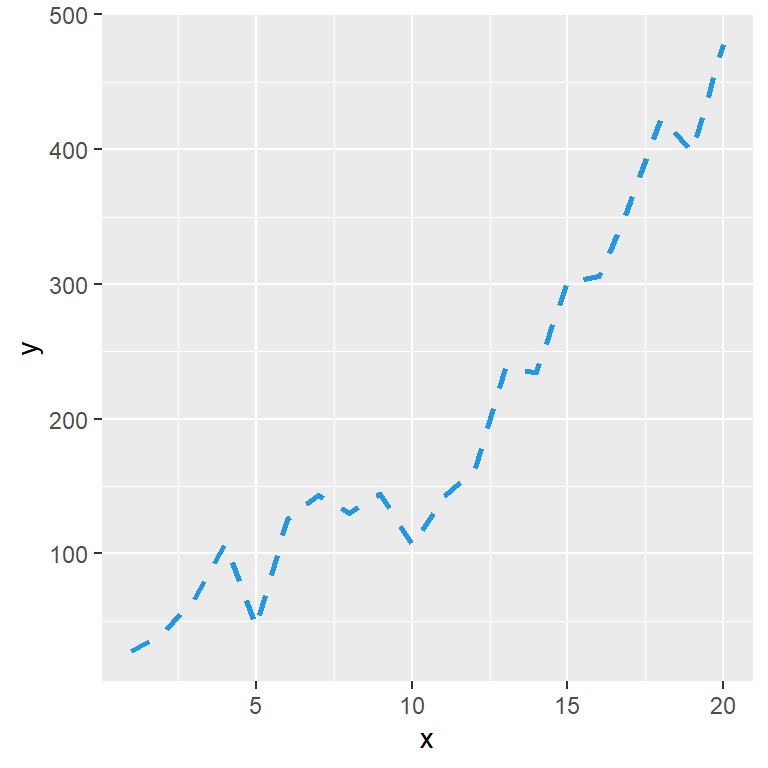# Line graph in ggplot2

## Basic line charts with `geom_line`

Given a data frame with a numerical variable `x` and a numerical variable `y` representing the value for each observation it is possible to create a line chart in ggplot with `geom_line` as follows.

``````# install.packages("ggplot2")
library(ggplot2)

# Data
set.seed(1)
x <- 1:20
y <- x ^ 2 + runif(20, 0, 100)
df <- data.frame(x = x, y = y)

ggplot(df, aes(x = x, y = y)) +
geom_line()``````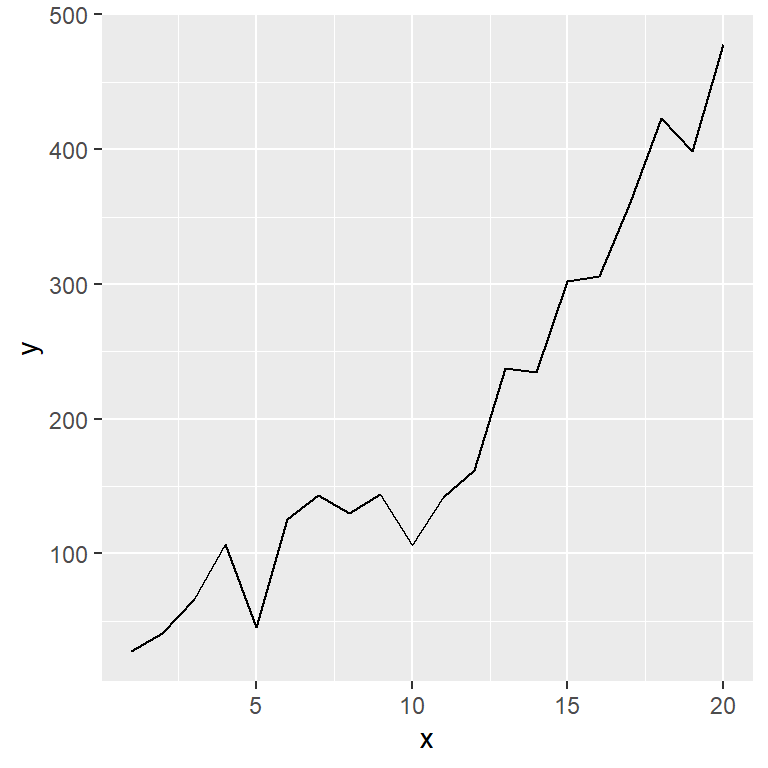If you add `geom_point` to the plot a point will be added for each observation.

``````# install.packages("ggplot2")
library(ggplot2)

# Data
set.seed(1)
x <- 1:20
y <- x ^ 2 + runif(20, 0, 100)
df <- data.frame(x = x, y = y)

ggplot(df, aes(x = x, y = y)) +
geom_line() +
geom_point()``````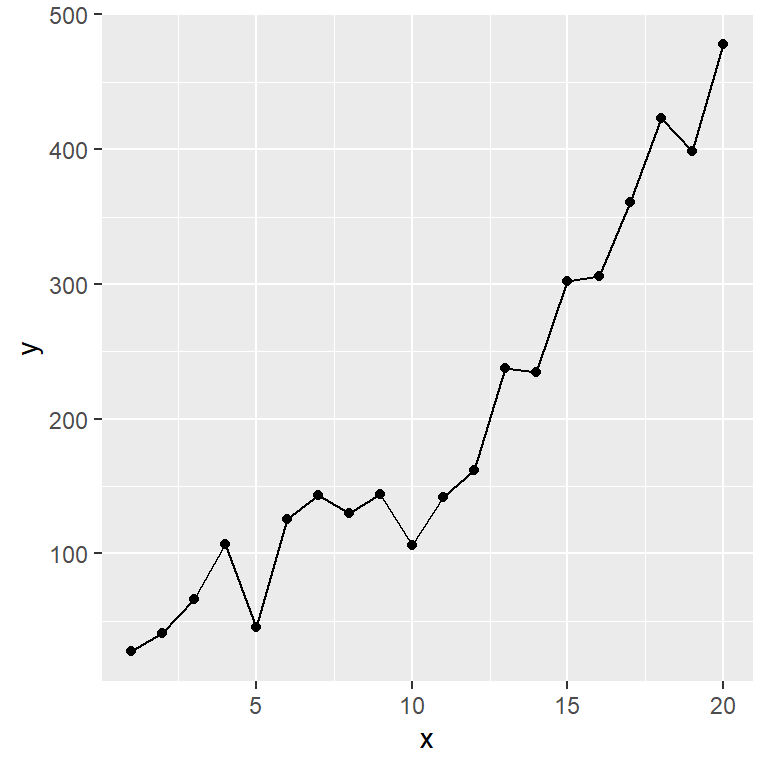Line as an arrow

If your data represents a trend or a path you can end the line with an arrow, passing the `arrow` funtion to the `arrow` argument. Type `?arrow` for additional arguments.

``````# install.packages("ggplot2")
library(ggplot2)

# Data
set.seed(1)
x <- 1:20
y <- x ^ 2 + runif(20, 0, 100)
df <- data.frame(x = x, y = y)

ggplot(df, aes(x = x, y = y)) +
geom_line(arrow = arrow())``````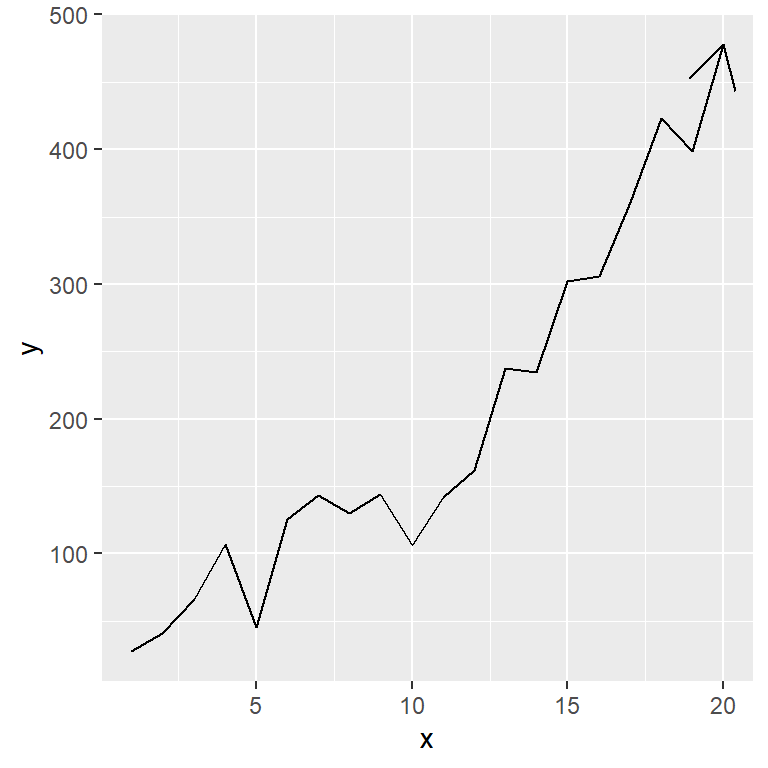## Stairstep plots with `geom_step`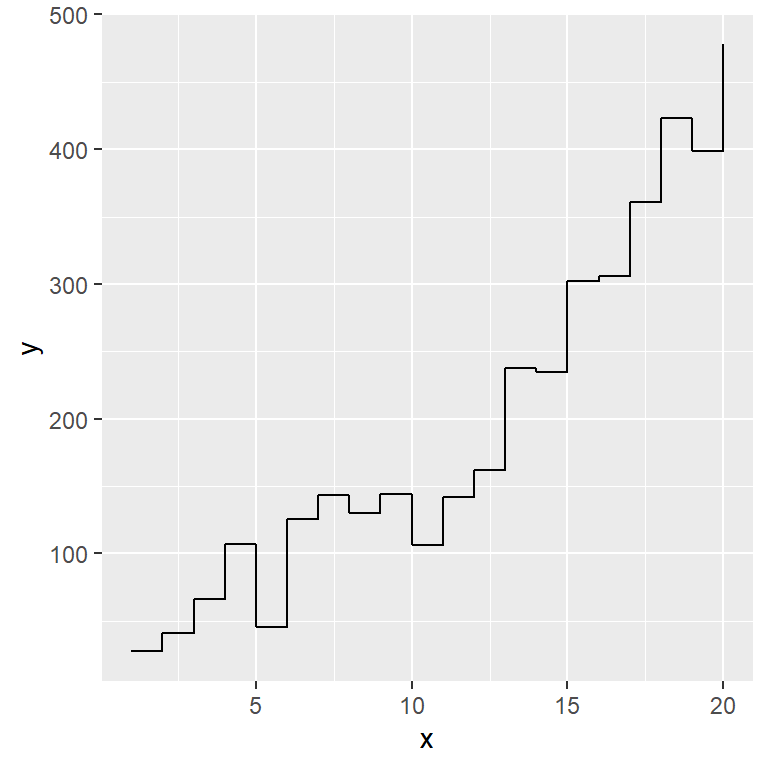First horizontal

An alternative geom is `geom_step`, which will create a stairstep plot where the first line is horizontal by default.

``````# install.packages("ggplot2")
library(ggplot2)

# Data
set.seed(1)
x <- 1:20
y <- x ^ 2 + runif(20, 0, 100)
df <- data.frame(x = x, y = y)

ggplot(df, aes(x = x, y = y)) +
geom_step()``````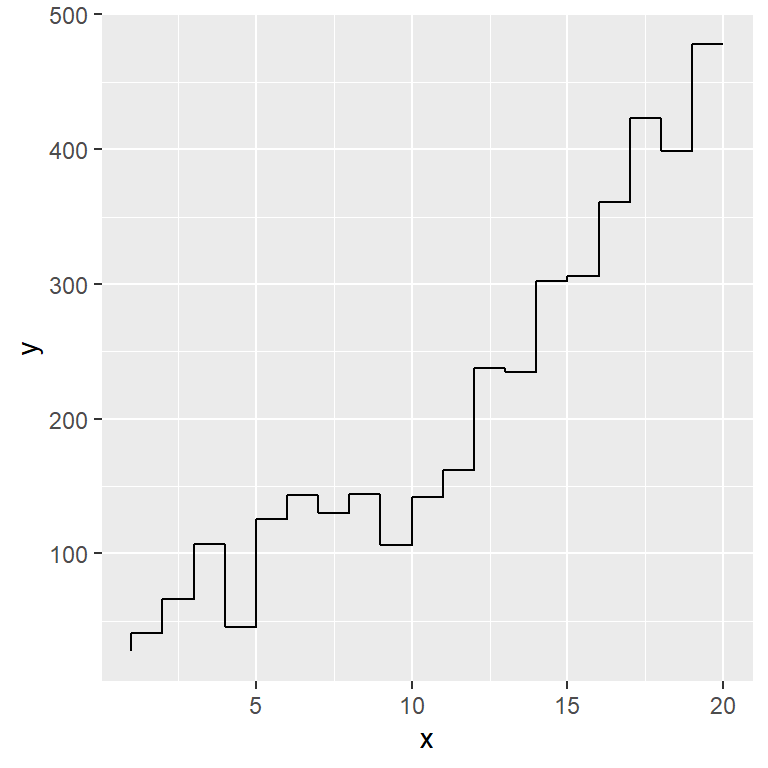First vertical

To set the first line vertical pass the string `"vh"` to the `direction` argument of the geom.

``````# install.packages("ggplot2")
library(ggplot2)

# Data
set.seed(1)
x <- 1:20
y <- x ^ 2 + runif(20, 0, 100)
df <- data.frame(x = x, y = y)

ggplot(df, aes(x = x, y = y)) +
geom_step(direction = "vh")``````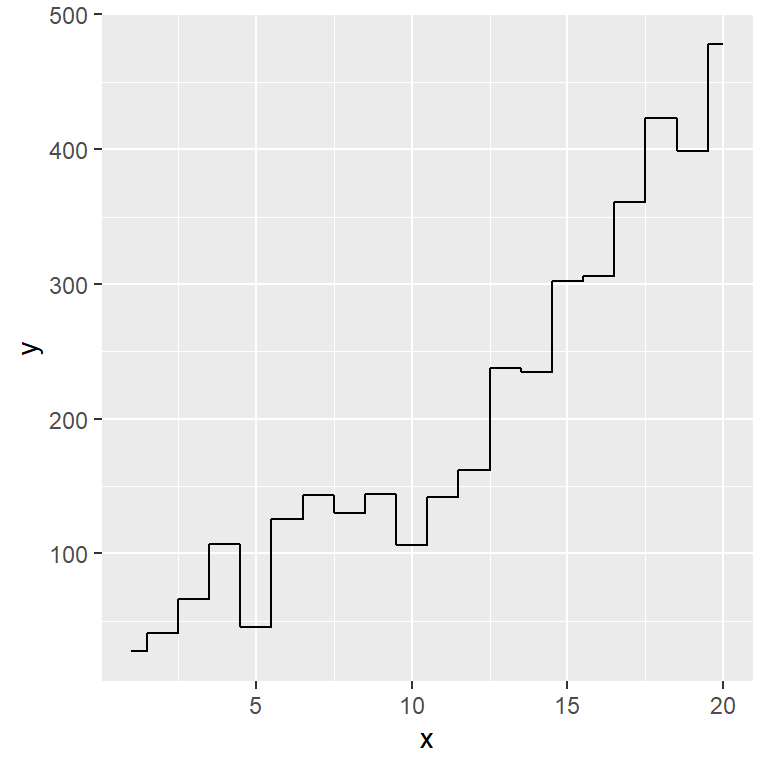Step half-way between values

Other possible option is setting `"mid"`, for step half-way between adjacent X-axis values.

``````# install.packages("ggplot2")
library(ggplot2)

# Data
set.seed(1)
x <- 1:20
y <- x ^ 2 + runif(20, 0, 100)
df <- data.frame(x = x, y = y)

ggplot(df, aes(x = x, y = y)) +
geom_step(direction = "mid")``````

## Line colors and styles

The `color`, `lwd` and `linetype` arguments can be used to modify the color, width and the type of the lines, respectively.

``````# install.packages("ggplot2")
library(ggplot2)

# Data
set.seed(1)
x <- 1:20
y <- x ^ 2 + runif(20, 0, 100)
df <- data.frame(x = x, y = y)

ggplot(df, aes(x = x, y = y)) +
geom_line(color = 4,    # Color of the line
lwd = 1,      # Width of the line
linetype = 1) # Line type``````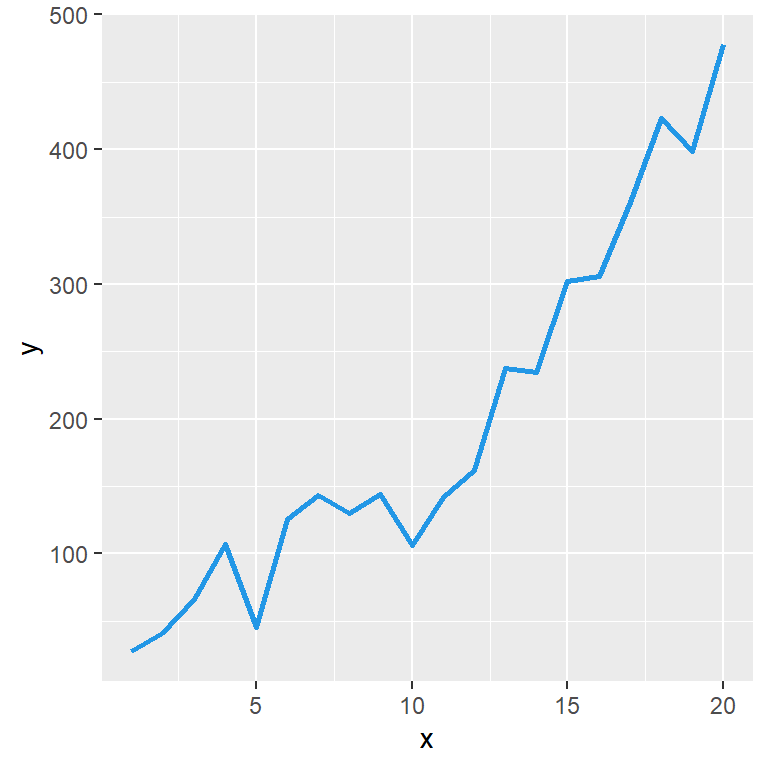Note that the line type can be set with numbers (0 to 6), with texts or with string patterns.

``````# install.packages("ggplot2")
library(ggplot2)

# Data
set.seed(1)
x <- 1:20
y <- x ^ 2 + runif(20, 0, 100)
df <- data.frame(x = x, y = y)

ggplot(df, aes(x = x, y = y)) +
geom_line(color = 4,
lwd = 1,
linetype = "dashed") ``````